## Intro

This course includes both example code and exercises for you to learn the concepts.
library, you probably don’t have it, and should use the `install.packages()` function to load them.

## ggplot2 basics

ggplot2 was devloped by Hadley Wickham, a notable R programmer and contributor to Rstudio.
The project stable, and should be available for the long term.
Use http://docs.ggplot2.org/current/ as a reference.
http://www.r-bloggers.com/ is a collection of recent articles on R.
To save a plot, use `ggsave`

``````#source("http://bib.umassmed.edu/~wespisea/rCourse/adamGgplot2/answers.R")
library(ggplot2)
library(plyr)
library(grid)
library(reshape2)
library(gridExtra)
library(MASS)
library(HistData)``````

## Scatter Plots

To create a scatter plot using qplot you would use:

``qplot(data=iris,x=Sepal.Width,y=Sepal.Length,geom="point")``

For ggplot2, the command is:

``ggplot(iris,aes(x=Sepal.Width,y=Sepal.Length)) + geom_point() ``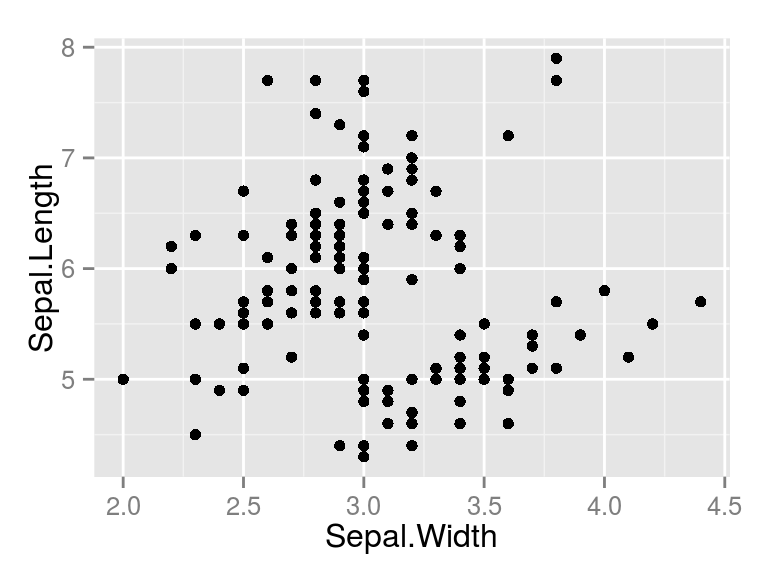### Question 1

Use the women dataset to plot average heights and weights for american women.
First, check the contents of the dataset using `str(women)` and use `help(women)` to learn about the data. Check the answer by `cat(answer1)`.

## Aesthetic Mappings

we can map: color, shape to data.frames or arbritrary values

``ggplot(iris,aes(x=Sepal.Width,y=Sepal.Length,shape=Species)) + geom_point()``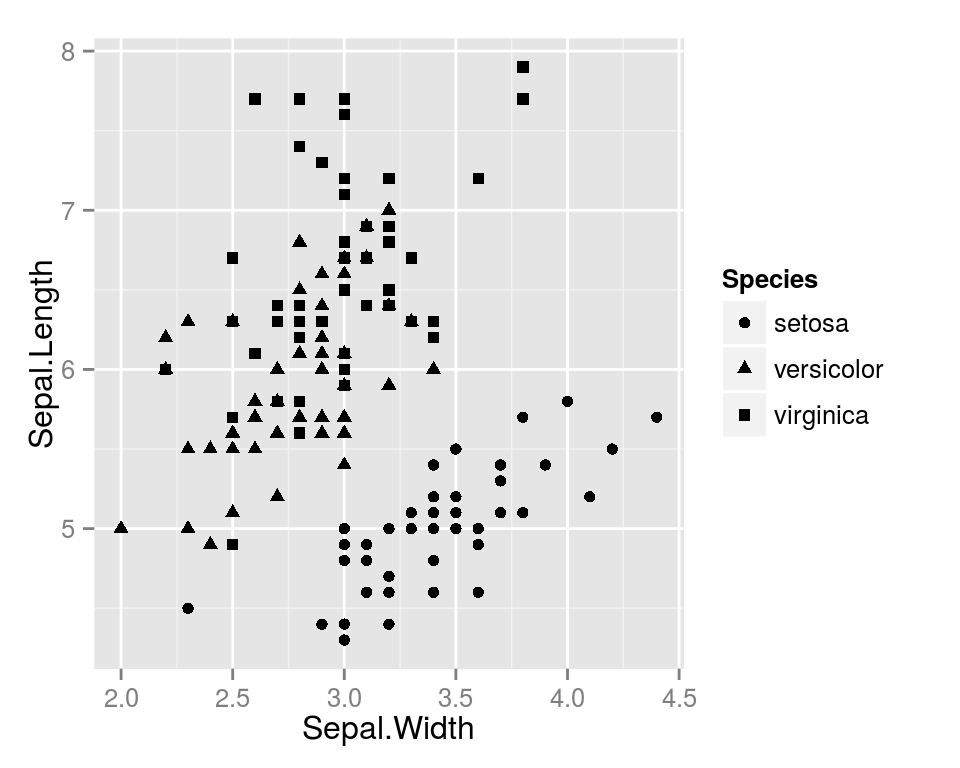``ggplot(iris,aes(x=Sepal.Width,y=Sepal.Length,color=Species)) + geom_point()``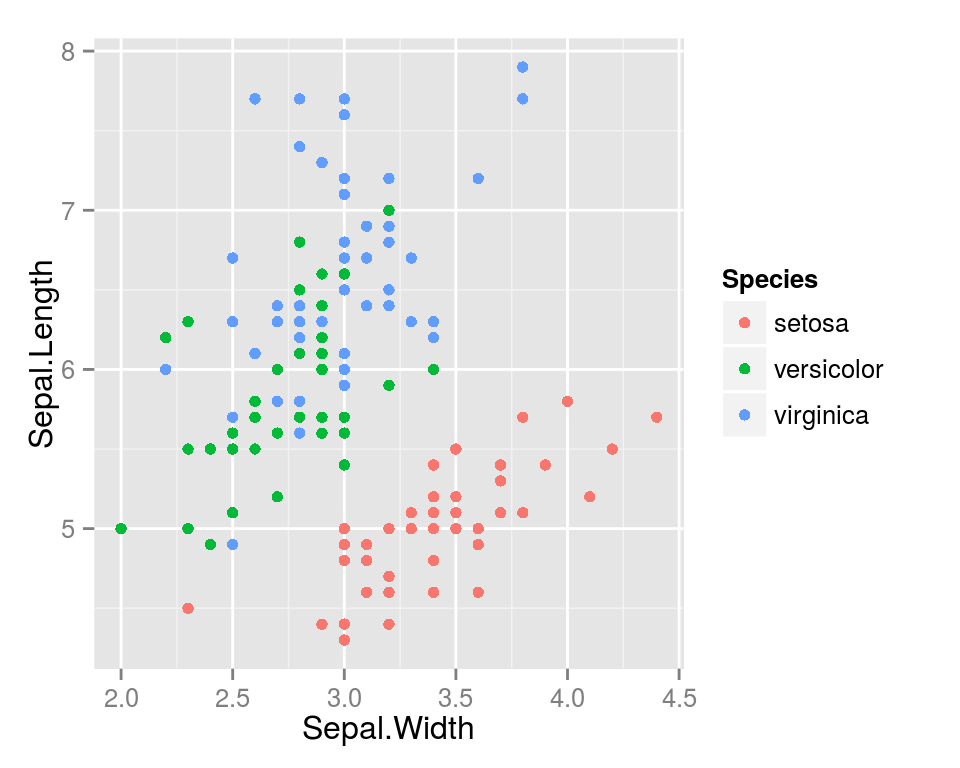### Question 2

Using the `birthwt` dataset, what is the distribution of age vs. weight?
By coloring the points, is low birth weight associated with either variable?
Check the answer by `cat(answer2)`.

#### Set aesthetics to fixed values:

``ggplot(iris,aes(x=Sepal.Width,y=Sepal.Length)) + geom_point(color="red",size=4)  ``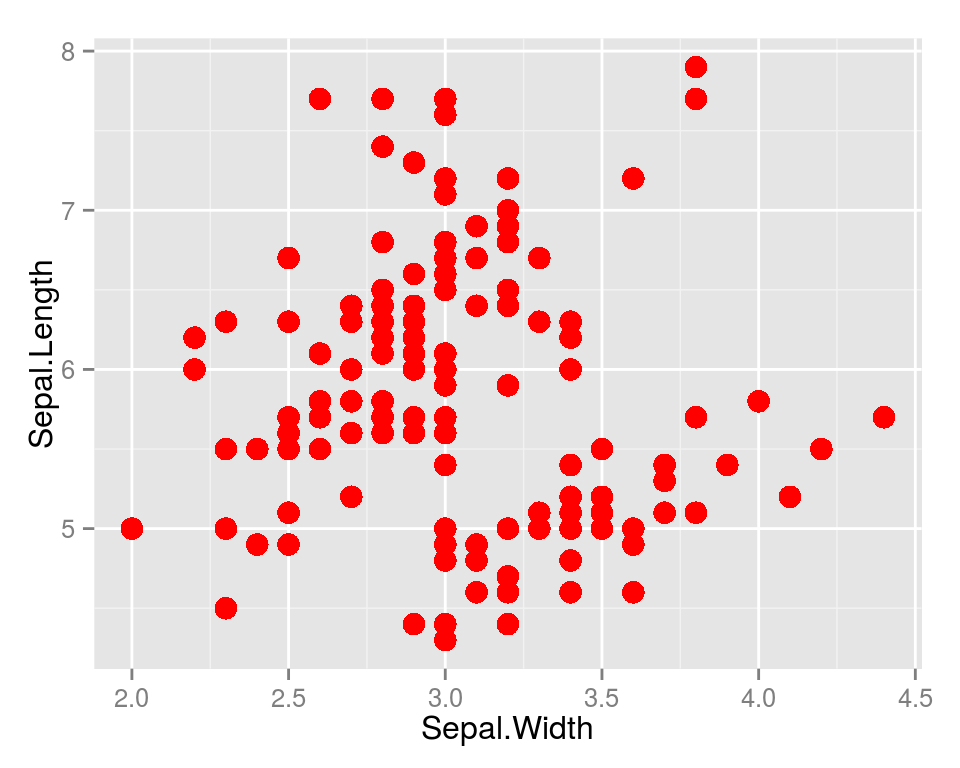``ggplot(diamonds,aes(x=carat,y=price))+ geom_point(alpha=I(0.4))``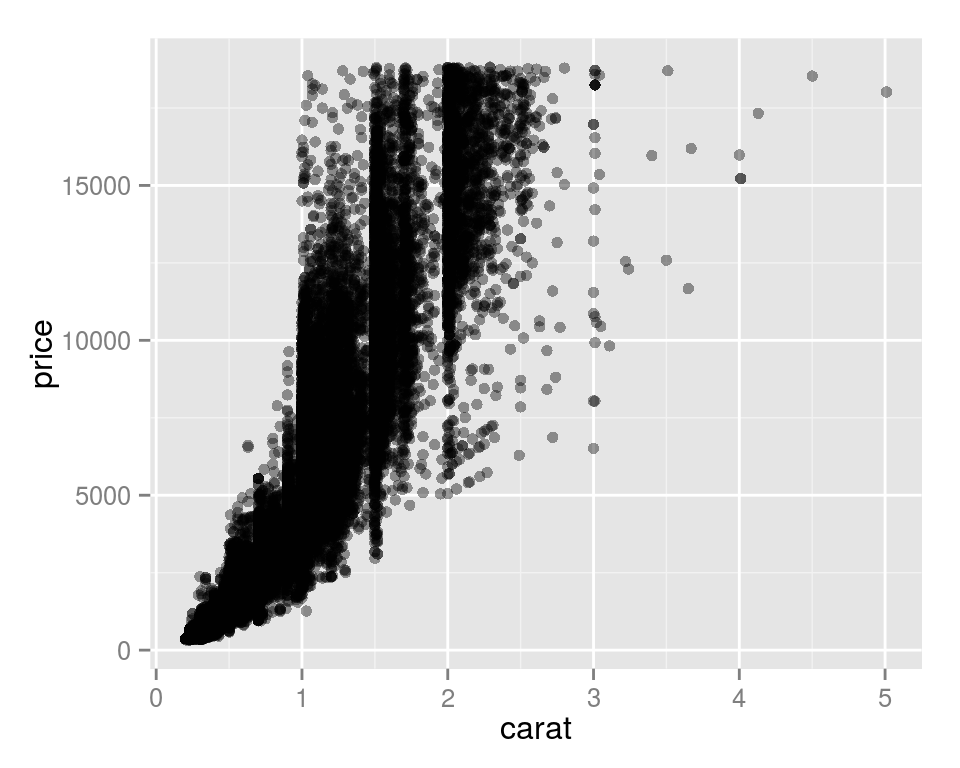``ggplot(diamonds,aes(x=carat,y=price))+ geom_point(alpha=I(0.1))``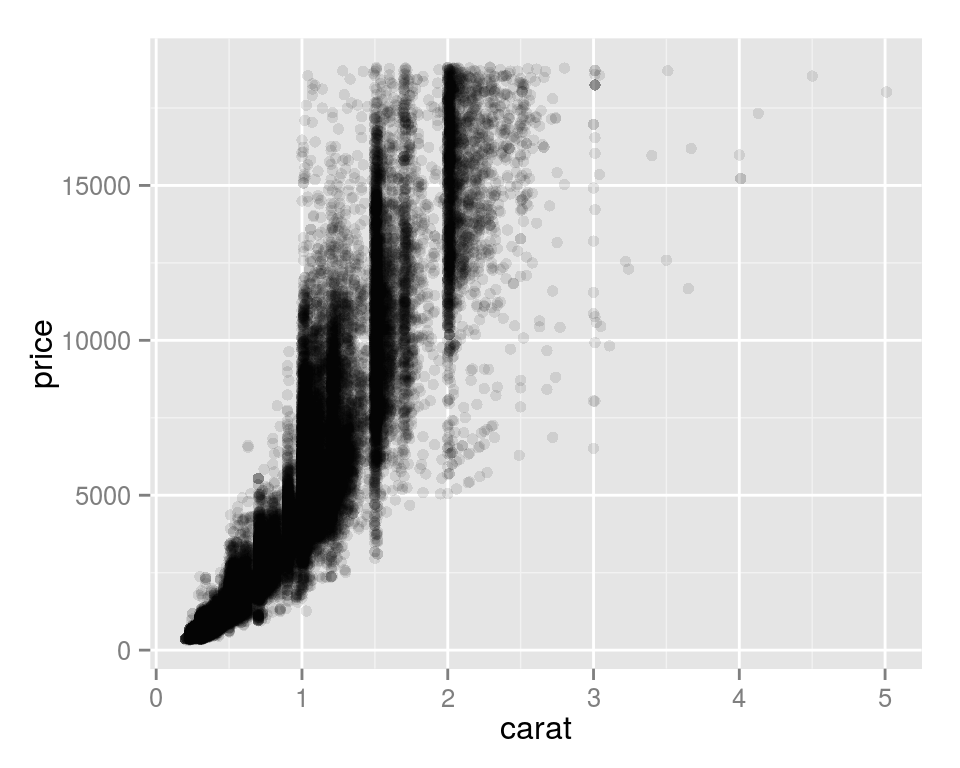#### Layered geoms

``````ggplot(iris,aes(x=Sepal.Width,y=Sepal.Length,color=Species,shape=Species)) +
geom_point(size=4) +
geom_point(size=2,color="grey")``````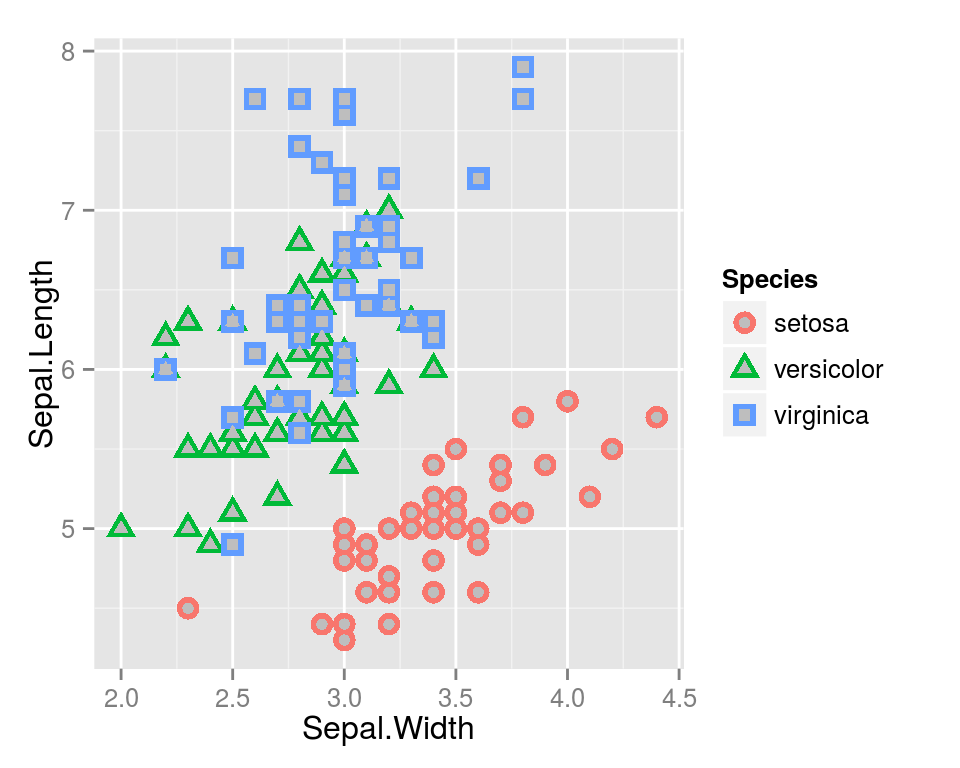## Line Plot

note, the aesthetic mappings work the same

``ggplot(economics,aes(x=date,y=uempmed))+geom_line()``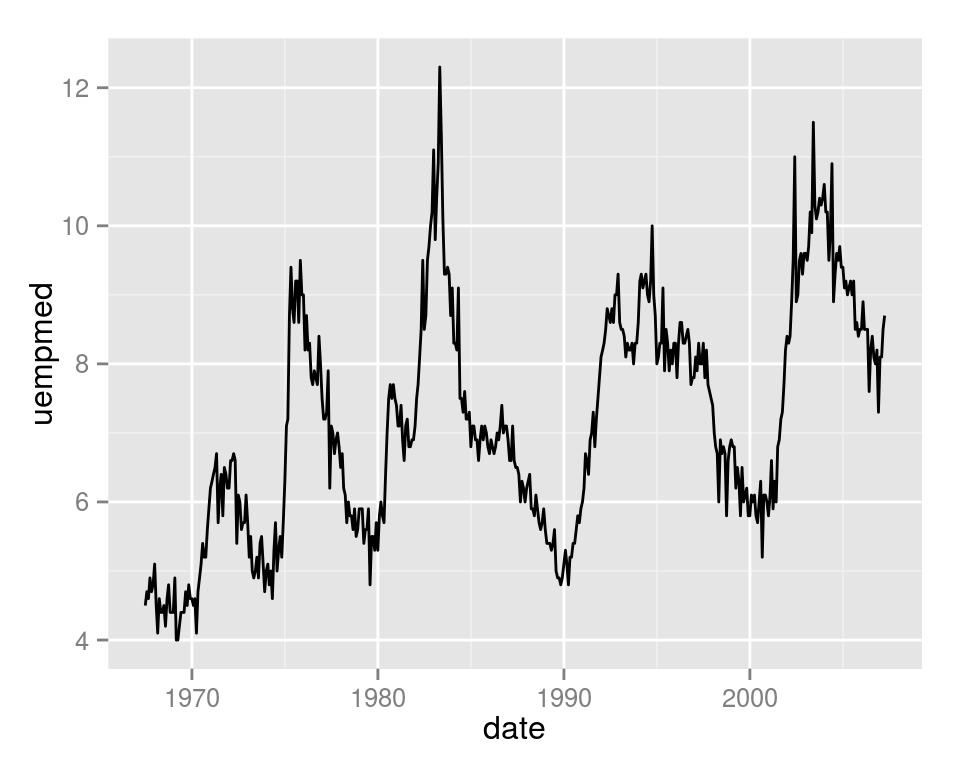### Question 3

plot the number of Typhoid Fever deaths per year using the epi dataset. If you are stuck, take a look at `cat(hint3)` and `cat(hint3.1)` for the data transforms. Check the answer by `cat(answer3)`.

#### Add labels and a title:

``````ggplot(economics,aes(x=date,y=uempmed))+ geom_line() +
xlab("Time") +
ylab("Unemployment Rate") +
ggtitle("Unemployment Rate vs Time\n1967-2007")``````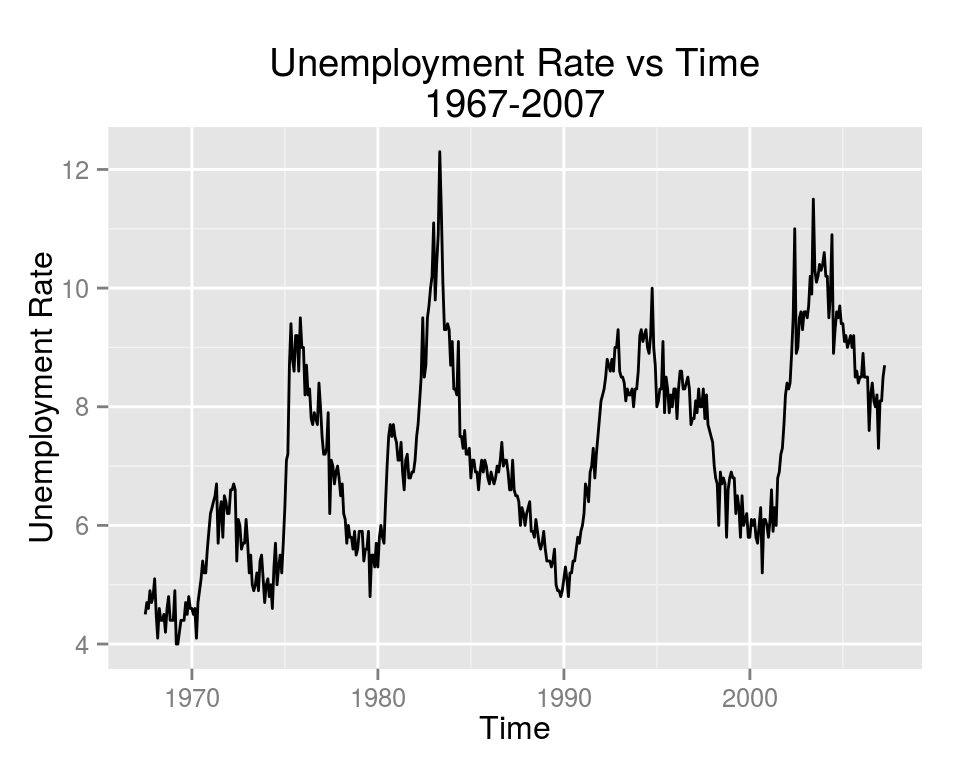### Question 4

Add labels and a title to the previous plot. Example solution: `cat(answer4)`.

## Bar Plot

``ggplot(mtcars,aes(x=cyl))+geom_bar(binwidth=1) ``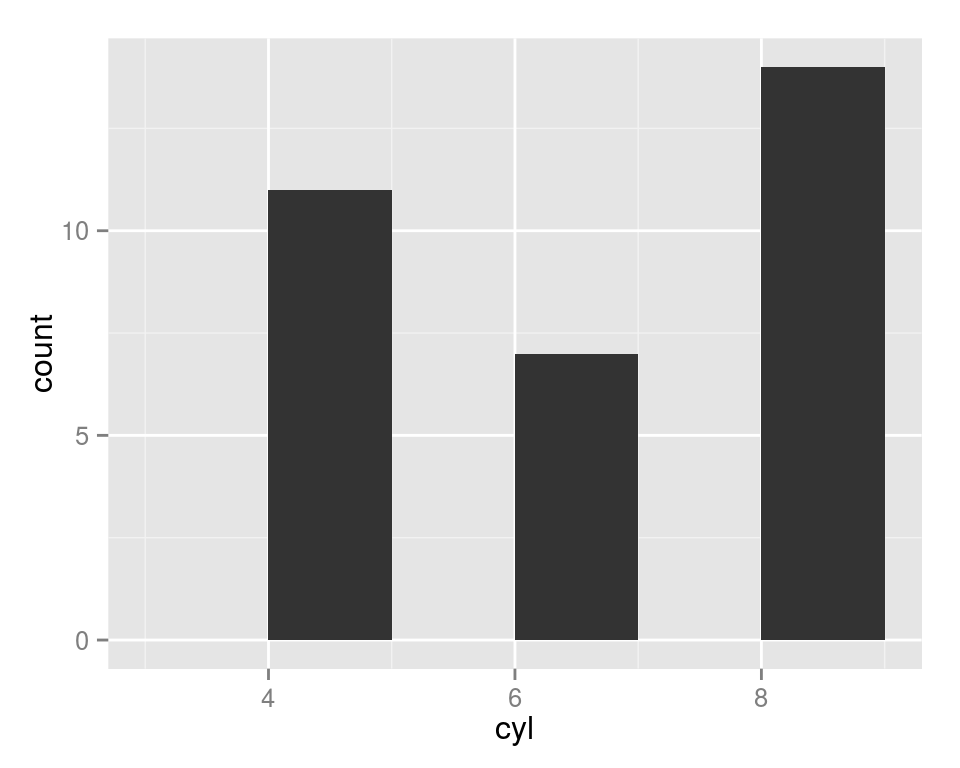#### use factor instead of numerical datatypes

``ggplot(mtcars,aes(x=factor(cyl)))+geom_bar()``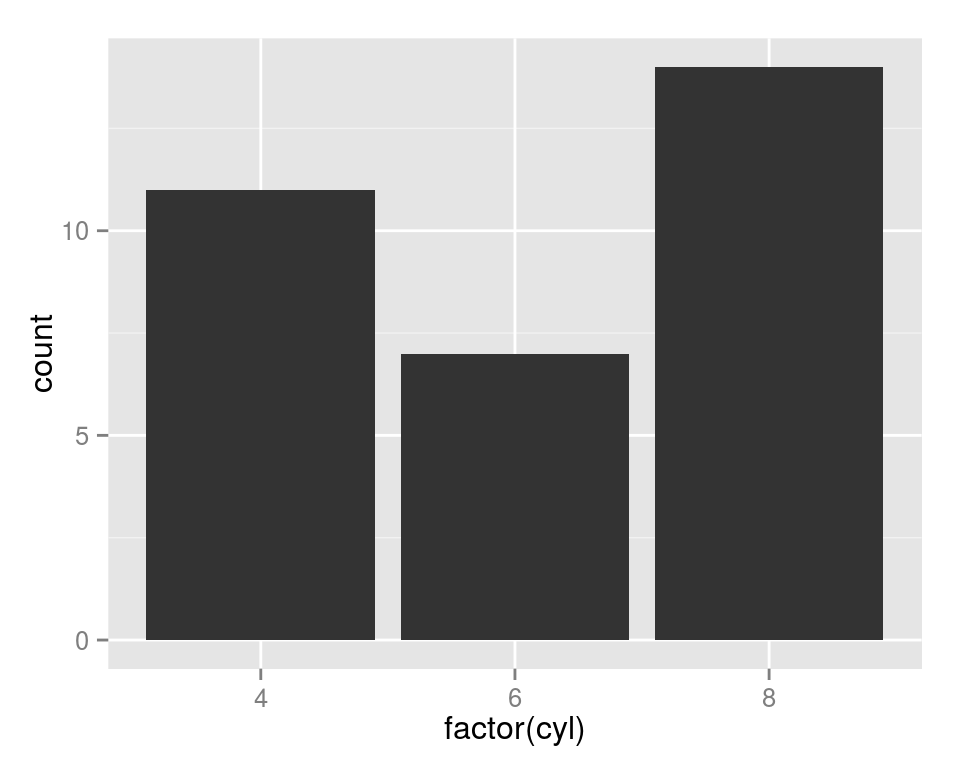#### color the bars by type

``ggplot(mtcars,aes(x=factor(cyl),fill=factor(gear)))+geom_bar()``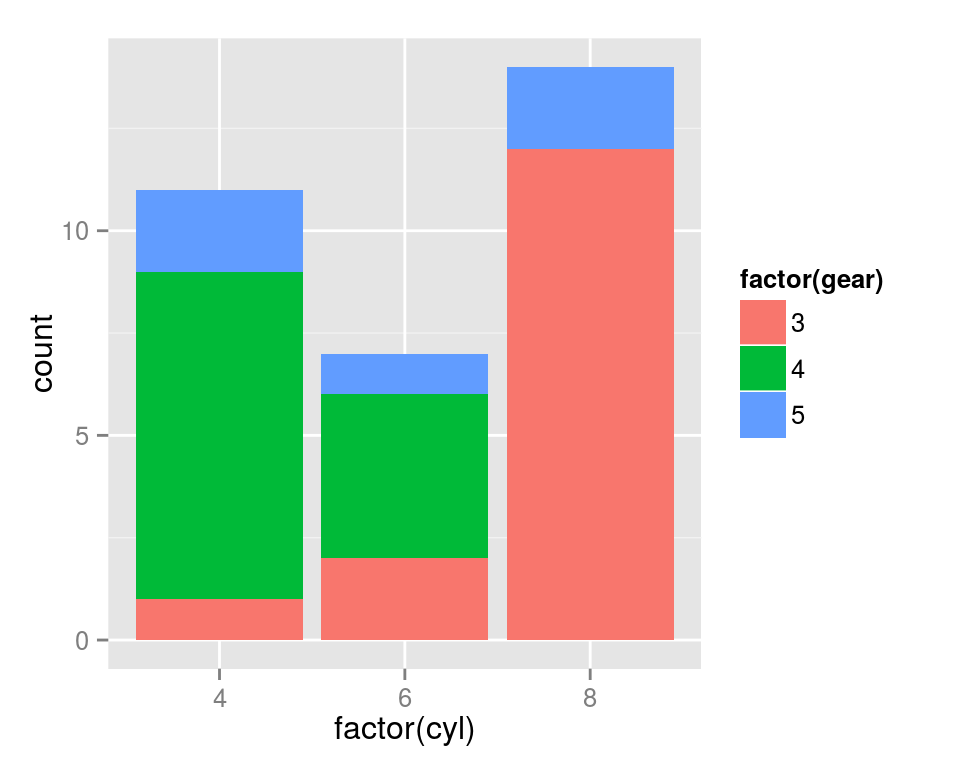#### another, better example:

``ggplot(diamonds, aes(clarity, fill=cut)) + geom_bar()``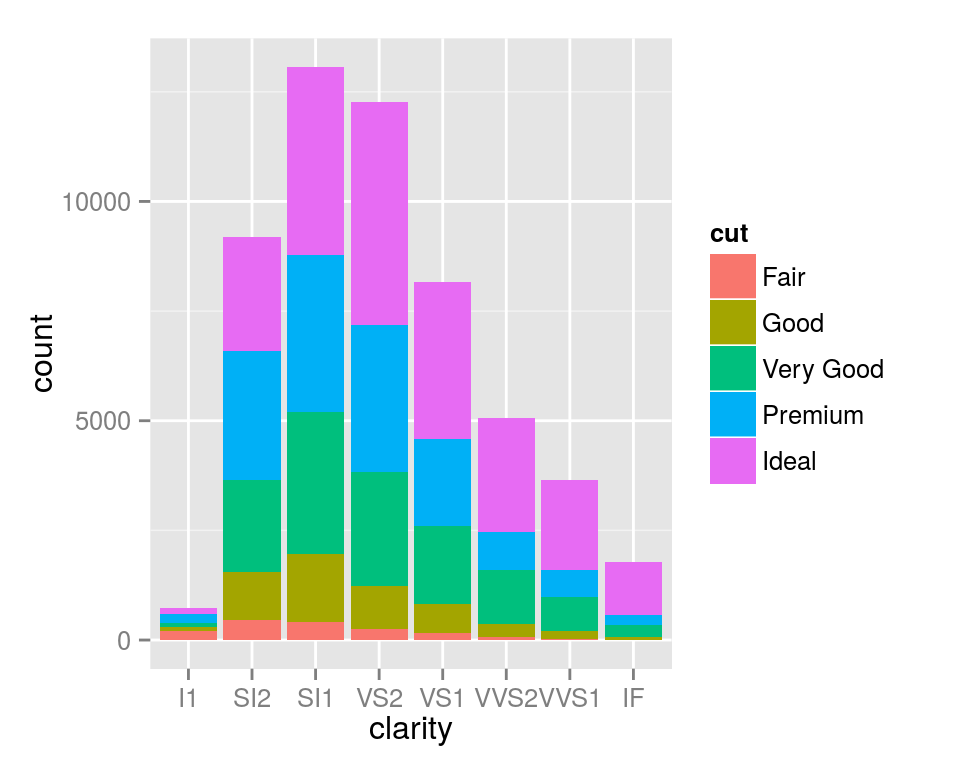## Question 5

What is the distribution of cases of typhoid per week? What about per year?
See `cat(answer5)` and `cat(answer5.1)`

### Density Plot

``ggplot(movies, aes(length))+geom_density()``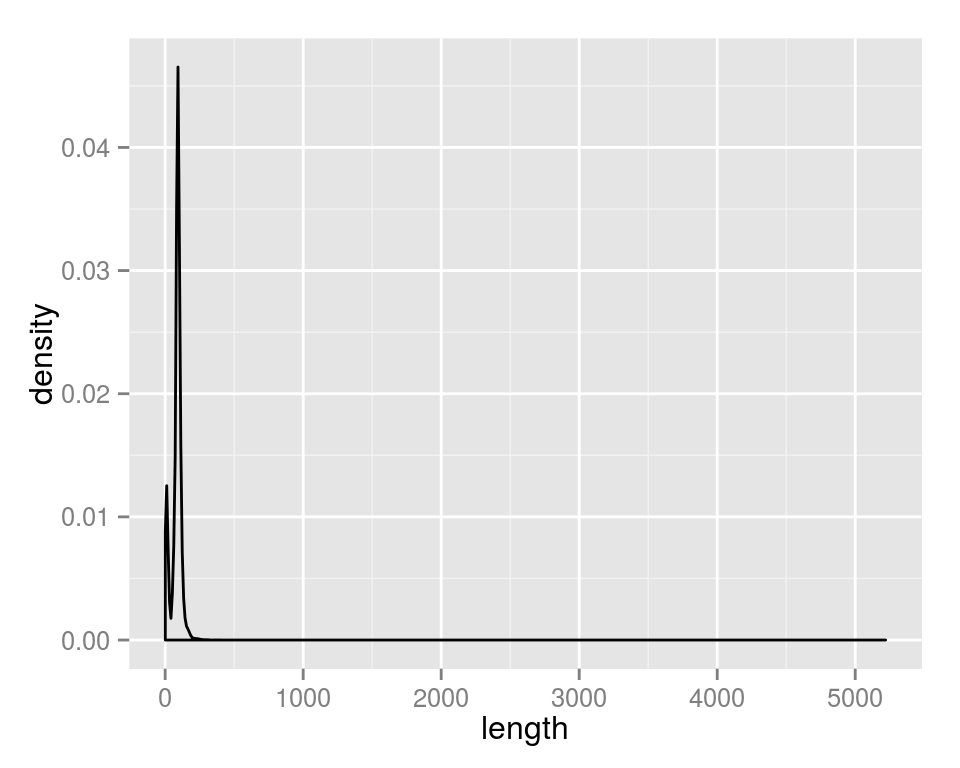#### Adjust x and y limits

we need to “zoom in” to get a resonable view create distributions of short vs. non-short movies:

``ggplot(movies, aes(x=length,fill=factor(Short)))+geom_density() + xlim(0,200)``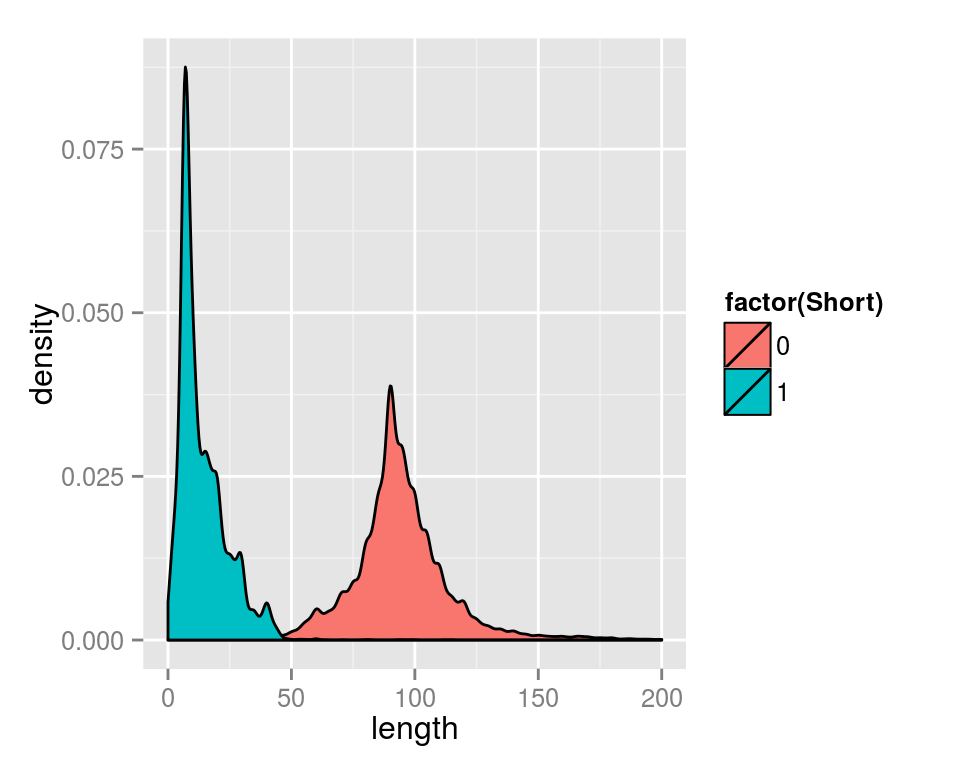#### Set transperancy of distro

``ggplot(iris, aes(x=Sepal.Width,fill=Species))+geom_density(alpha=I(0.4))``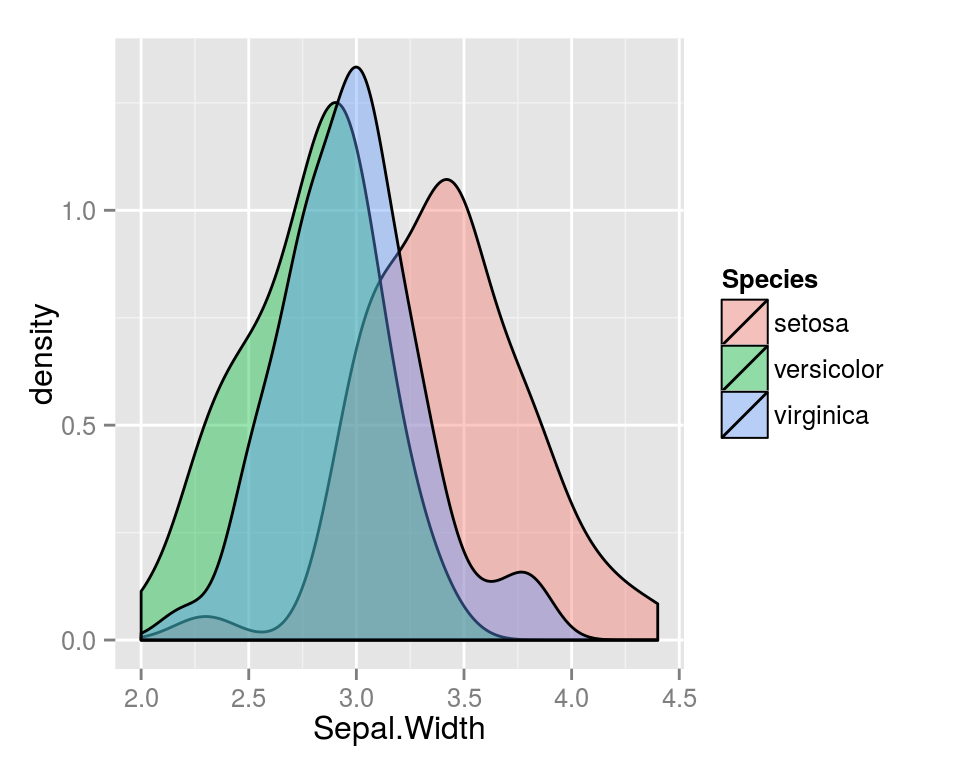### Question 6a

High glycosaminoglycans (GAGs) urine levels can progressively damage tissue,
and high levels are indictitive of inherited disease.Using the `GAGurine` dataset
from the MASS package, what is the distribution of GAG assay values?
Check the answer by `cat(answer6a)`.

### Question 6b

What’s the difference in GAG distribution between children under 2 and
all other children?
see `cat(hint6b)` for data transform.
Check the answer by `cat(answer6)`.

## Boxplot

``````ggplot(movies[movies\$year > 1990,],aes(x=factor(year),y=rating)) +
geom_boxplot()``````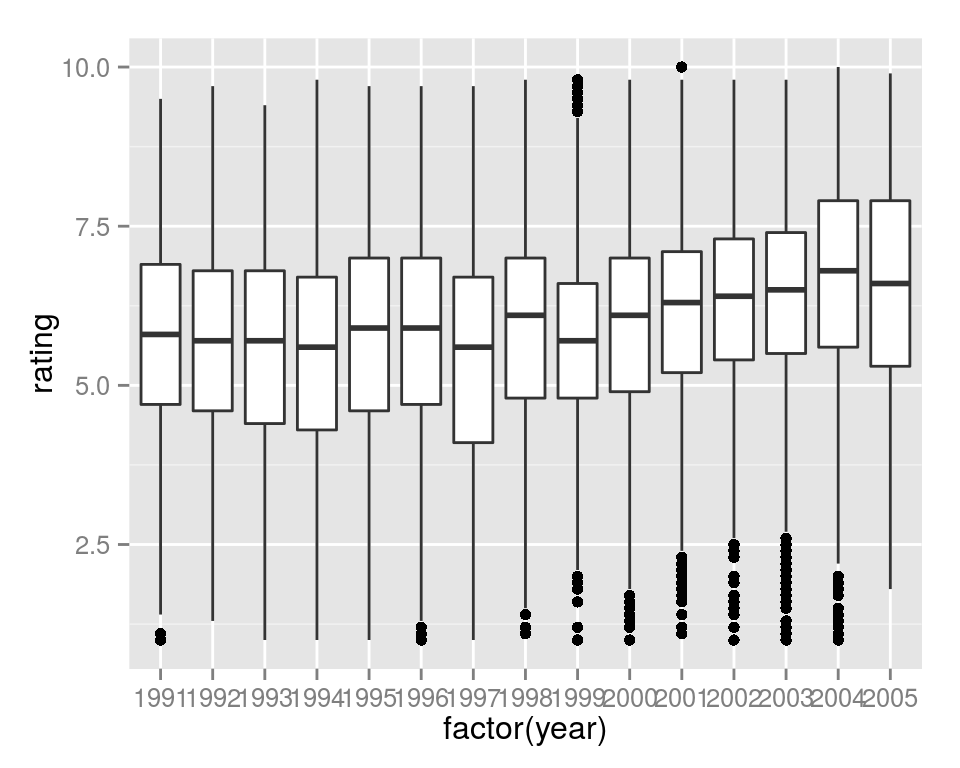``````ggplot(movies[movies\$year > 1990,],aes(x=factor(year),y=rating,fill=factor(Short))) +
geom_boxplot()``````